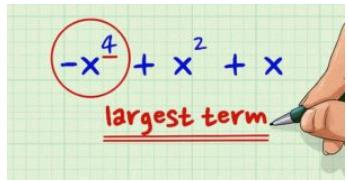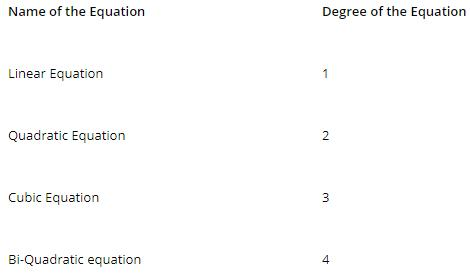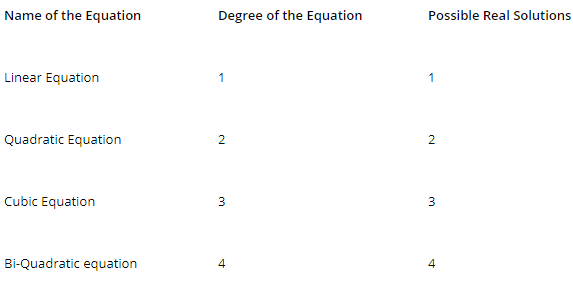Courses

# Degree of Polynomial Mathematics Notes | EduRev

## Algebra for IIT JAM Mathematics

Created by: Veda Institute

## Mathematics : Degree of Polynomial Mathematics Notes | EduRev

The document Degree of Polynomial Mathematics Notes | EduRev is a part of the Mathematics Course Algebra for IIT JAM Mathematics.
All you need of Mathematics at this link: MathematicsPolynomial in One Variable

The degree of polynomials in one variable is the highest power of the variable in the algebraic expression. For example, in the following equation: x2+2x+4. The degree of the equation is 2 .i.e. the highest power of variable in the equation.

Multivariable polynomial

For a multivariable polynomial, it the highest sum of powers of different variables in any of the terms in the expression. Take following example, x5+3x4y+2xy3+4y2-2y+1. It is a multivariable polynomial in x and y, and the degree of the polynomial is 5 – as you can see the degree in the terms x5 is 5, x4y it is also 5 (4+1) and so the highest degree among these individual terms is 5.

A polynomial of two variable x and y, like axrys is the algebraic sum of several terms of the prior mentioned form, where r and s are possible integers. Here, the degree of the polynomial is r+s where r and s are whole numbers.

Note: Exponents of variables of a polynomial .i.e. degree of polynomials should be whole numbers.

How to find the Degree of a Polynomial?

There are 4 simple steps are present to find the degree of a polynomial:-

Example: 6x5+8x3+3x5+3x2+4+2x+4

• Step 1: Combine all the like terms that are the terms of the variable terms
(6x5+3x5)+8x3+3x2+2x+(4+4)
• Step 2: Ignore all the coefficients
x5+x3+x2+x+x0
• Step 3: Arrange the variable in descending order of their powers
x5+x3+x2+x+x0
• Step 4: The largest power of the variable is the degree of the polynomial
deg(x5+x3+x2+x+x0) = 5

Classification Based on the Degree of the Equation

Based on the degree, the equation can be linear, quadratic, cubic, and bi-quadratic, and the list goes on.Importance of Degree of polynomial

Case of Homogeneous Polynomial

The degree of terms is a major deciding factor whether an equation is homogeneous or not. A polynomial of more that one variable is said to be homogeneous if the degree of each term is the same. For example, 2x7+5x5y2-3x4y3+4x2y5 is a homogeneous polynomial of degree 7 in x and y.
Relation of Degree of Polynomials with Zeroes of Equation

Theorem 1: A polynomial f(x) of the nth degree cannot vanish for more than n values of x unless all its coefficients are zero.The above table shows possible real zeros /solutions; actual real solutions can be less than the degree of the equation.

Note: A constant polynomial is that whose value remains the same. It contains no variables. The power of the constant polynomial is Zero. Well, you can write any constant with a variable having an exponential power of zero. If the constant term = 4, then the polynomial form is given by f(x)= 4x0

Before going to start other sections of Polynomials, try to solve the below-given question.

A Question for You

Question: Find the degree of polynomial x3+4x5+5x4+2x2+x+5.

Solution: x3+4x5+5x4+2x2+x+5

=4x5+5x4+x3+2x2+x+5

=x5+x4+x3+x2+5
Degree of equation is the highest power of x in the given equation .i.e. 5.

Offer running on EduRev: Apply code STAYHOME200 to get INR 200 off on our premium plan EduRev Infinity!

,

,

,

,

,

,

,

,

,

,

,

,

,

,

,

,

,

,

,

,

,

;